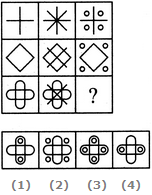# Non Verbal Reasoning - Figure Matrix - Discussion

### Discussion :: Figure Matrix - Section 1 (Q.No.2)

In each of the following questions, find out which of the answer figures (1), (2), (3) and (4) completes the figure matrix ?

2.

Select a suitable figure from the four alternatives that would complete the figure matrix.[A]. 1 [B]. 2 [C]. 3 [D]. 4

Explanation:

In each row, the second figure is obtained from the first figure by adding two mutually perpendicular line segments at the centre and the third figure is obtained from the first figure by adding four circles outside the main figure.

 Kifayat Ullah Shah said: (Jun 20, 2013) In a row just add four circles on its four side and ignore midlle figure.

 Name said: (Oct 8, 2016) Just put circles around first diagram.

 Laura said: (May 19, 2017) The 3rd option of the 1st picture, the circles are NOT outside the main figure but inside in the four quadrants. The 2nd figure, 3rd options the circles ARE outside the main figure. Therefore, I see the completion as option C to fulfill the inside-outside-inside.

 S.Ujwala said: (Jul 25, 2019) Thanks @Laura.